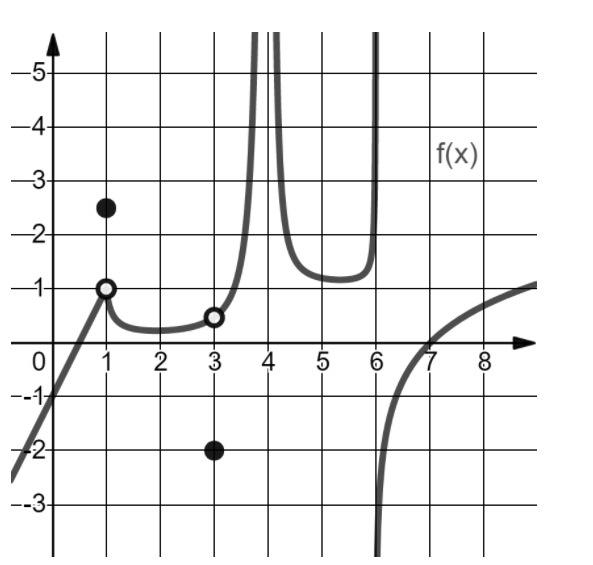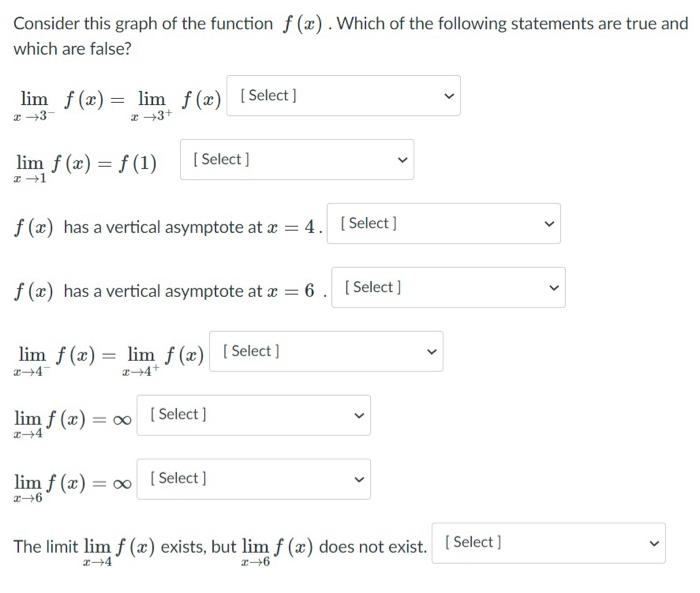# Question Solved1 Answerplease and thank you -5 -4 f(x) 3 -2 N 1 2 3 4 5 6 -00 0 ---1- 2 --3- Consider this graph of the function f(x). Which of the following statements are true and which are false? lim f(x) = lim f(x) [Select] 3- +3+ lim f(x) = f(1) Select) +1 > f(x) has a vertical asymptote at x = 4. Select] < f(x) has a vertical asymptote at x = 6. Select ] > lim f (x) = lim f(x) [Select ] 24+ < [ Select) lim f (x) = 0 2-4 lim f (x) = [Select] < The limit lim f (x) exists, but lim f (x) does not exist. (Select] 2-6Transcribed Image Text: -5 -4 f(x) 3 -2 N 1 2 3 4 5 6 -00 0 ---1- 2 --3- Consider this graph of the function f(x). Which of the following statements are true and which are false? lim f(x) = lim f(x) [Select] 3- +3+ lim f(x) = f(1) Select) +1 > f(x) has a vertical asymptote at x = 4. Select] < f(x) has a vertical asymptote at x = 6. Select ] > lim f (x) = lim f(x) [Select ] 24+ < [ Select) lim f (x) = 0 2-4 lim f (x) = [Select] < The limit lim f (x) exists, but lim f (x) does not exist. (Select] 2-6
More
Transcribed Image Text: -5 -4 f(x) 3 -2 N 1 2 3 4 5 6 -00 0 ---1- 2 --3- Consider this graph of the function f(x). Which of the following statements are true and which are false? lim f(x) = lim f(x) [Select] 3- +3+ lim f(x) = f(1) Select) +1 > f(x) has a vertical asymptote at x = 4. Select] < f(x) has a vertical asymptote at x = 6. Select ] > lim f (x) = lim f(x) [Select ] 24+ < [ Select) lim f (x) = 0 2-4 lim f (x) = [Select] < The limit lim f (x) exists, but lim f (x) does not exist. (Select] 2-6